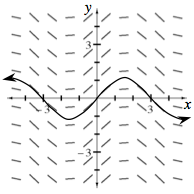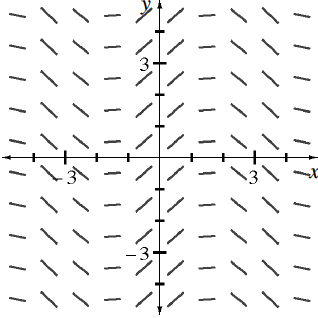### Home > APCALC > Chapter 7 > Lesson 7.3.4 > Problem7-143

7-143.

​​

Study the slope field for $\frac { d y } { d x }$ at right. Use it to visualize a possible function for $y$.

1. What type of functions are $y$ and $\frac { d y } { d x }$?

Imagine that the tangent lines were connected in a smooth way. Notice the oscillating pattern.

2. Sketch the particular solution of $y$ through the point $(0, 0)$ on your own set of axes.